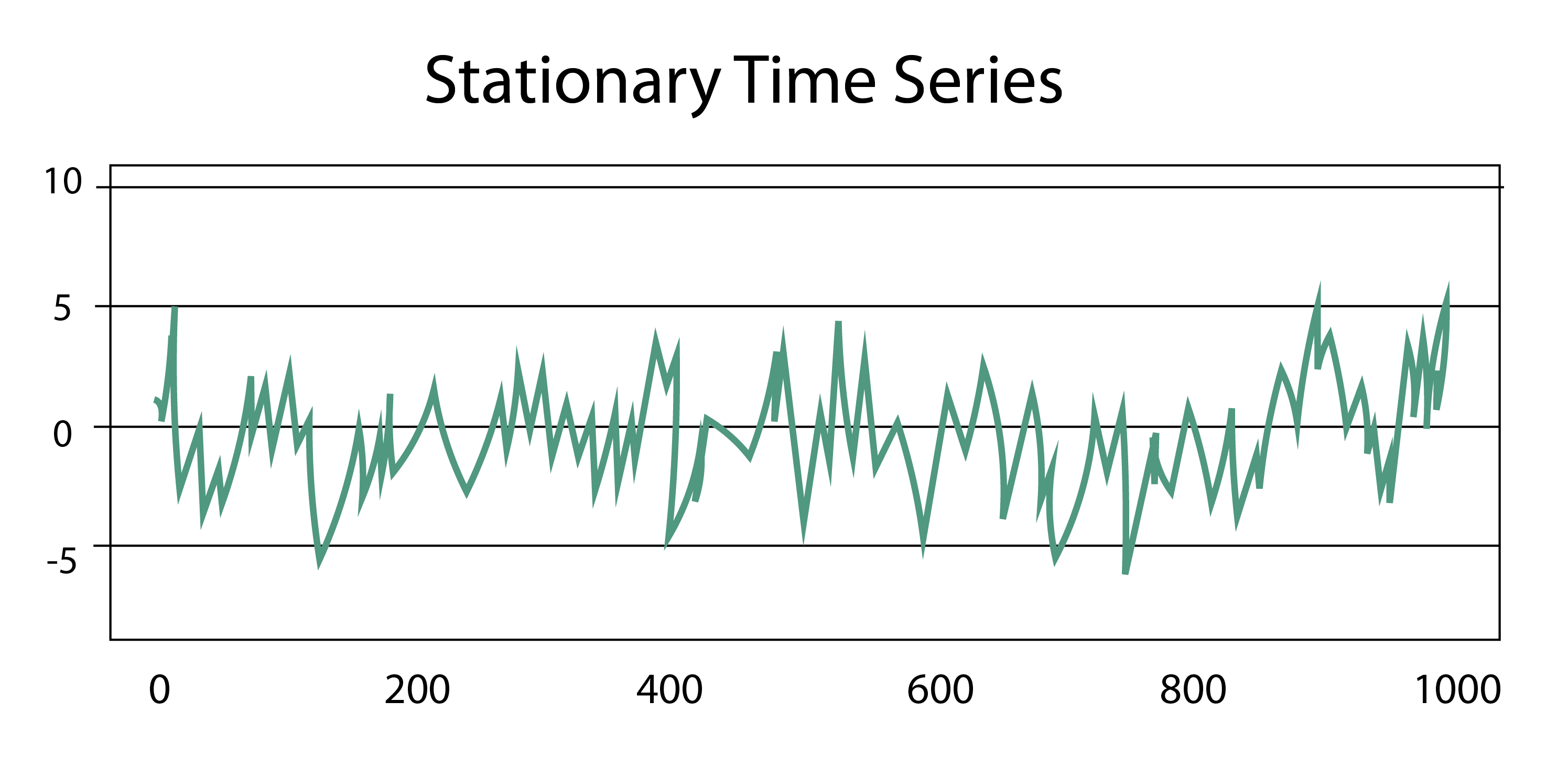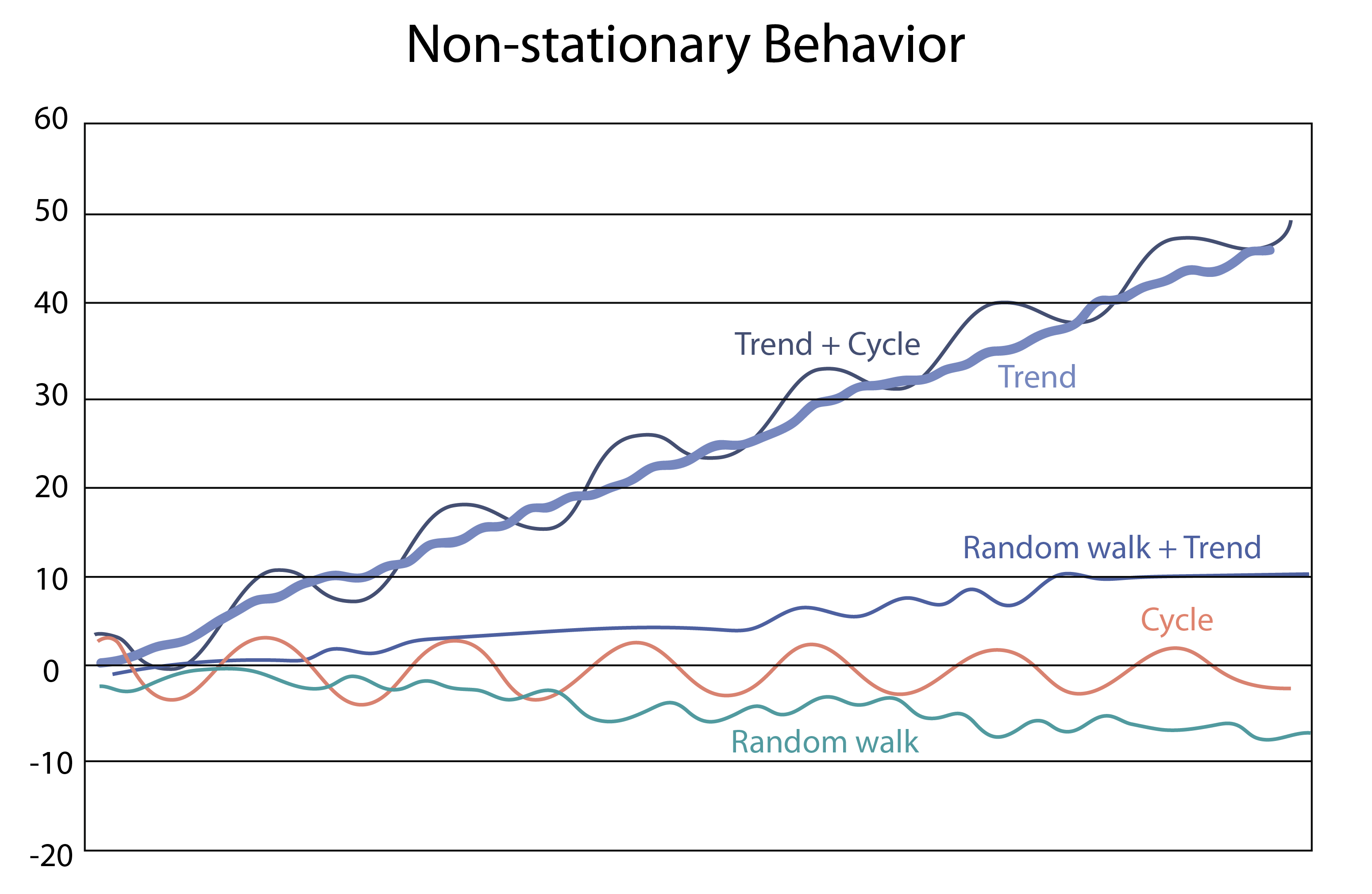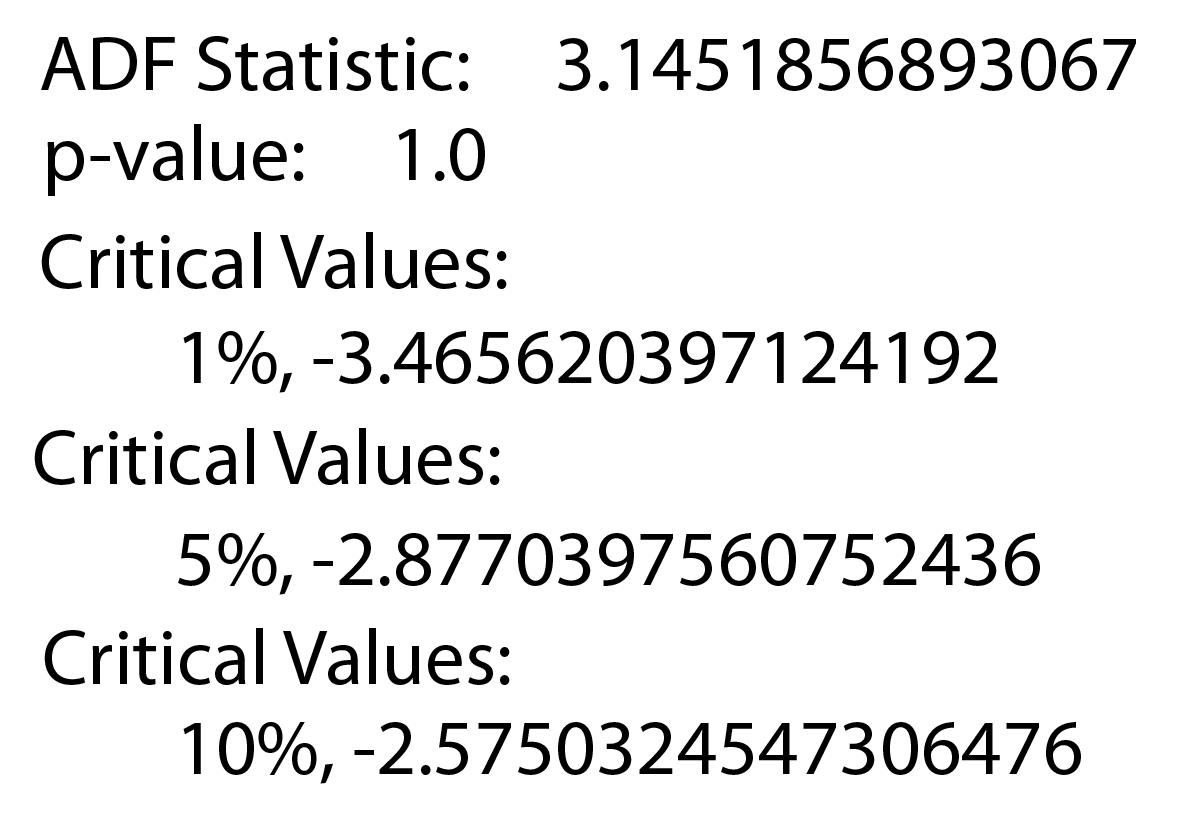Course Content

# Time Series Analysis

1. Time Series: Let's Start

2. Time Series Processing

3. Time Series Visualization

Time Series Analysis

##Stationarity

In time series analysis, stationarity refers to a feature of a stochastic process where the statistical properties of the process do not change over time. The process's mean, variance, and autocorrelation structure remain constant.

Stationary data example:And non-stationary data:Why is this feature important to us?

Its presence is a criterion in selecting models that you can use to predict the data. If the data is stationary, you can use diverse models ( Autoregression, Moving Average, Linear Regression, etc.). On the other hand, there are no universal models to predict non-stationary processes: some non-stationary time series can be converted to stationary via some mathematical manipulations (ARIMA model); other non-stationary time series can be forecasted using special types of neural networks.

Let's move on to checking the data for stationarity. This can be done using statistical tests called "Unit Root Tests". There are tests such as:

• Augmented Dickey-Fuller test;
• Kwiatkowski-Phillips-Schmidt-Shin;
• Philips Perron test.

Most often, we will use the Augmented Dickey-Fuller test (ADF), but you can take into account the results of other tests.

Using the `statsmodel` library, we will determine whether the data is stationary. This is done in a few lines of code:

How can we interpret the results obtained?The null hypothesis of this test is that the time series is non-stationary. If the p-value is less than some level (e.g., 0.05), we can reject the null hypothesis and conclude that the time series is stationary.

Let's look at the p-value above. It is equal to 1.0, which means that we cannot reject the null hypothesis, and our data is non-stationary. The null hypothesis would be false if the p-value were less than 0.05.

Check the `daily-total-female-births.csv` dataset for stationarity.
1. Import `adfuller` from `statsmodels.tsa.stattools`.
2. Read the `csv` file.
3. Perform the Augmented Dickey-Fuller test for the `"Births"` column of the `data`.